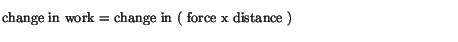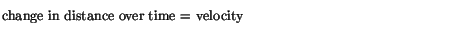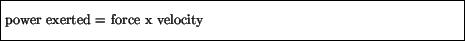Next: Kinetic Energy Up: Work and Energy Previous: Work

# Power

Note that the work done on an object is independent of the time taken to travel the distance covered. For example, suppose one lifts a 200 N weight off the floor a distance of 2 m. The work done in this case would be the product of the force exerted (the 200 N) times the distance covered (the 2 m), independent of how fast the weight was lifted. This is somewhat against our everyday idea of what work is; one might be tempted to say that one does more work'' if one lifts the weight faster. However, it is true that the same amount of work is done independently of the time taken; rather, it is the power in this case that is different. The power exerted by a force is defined asUsing the definition of work, we seeand assuming the force is constant, we find, usingthat the power can be expressed asThus, exerting a force at a larger velocity results in a greater power output, as we intuitively expect.

The units of power can be seen from its definition; they are the units of work (Joules) divided by the units of time (seconds). This combination of units (J/s) is called a Watt (W).

To get an idea of the sizes of the units involved, let us estimate the power output of a person running up a flight of stairs. Assuming the person has a weight of 600 N, and the flight of stairs is 4 m tall, the work done by the person is 600 x 4 = 2400 J. If the person does this in 3 s, the power output is 2400 / 3 = 800 W. One might be familiar with the British unit of power, the horsepower (hp), which was originally defined as the power rate at which an typical'' horse could do work, and is still the unit in which the power of car engines is given. The conversion between the two units is that 1 hp = 746 W. The 800 W of power thus exerted by this person is equivalent to 800 W x ( 1 hp / 746 W ) = 1.1 hp.Next: Kinetic Energy Up: Work and Energy Previous: Work
modtech@theory.uwinnipeg.ca
1999-09-29